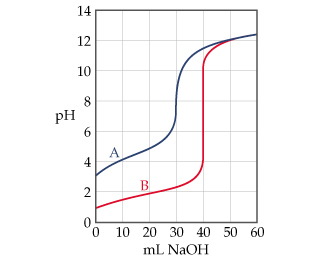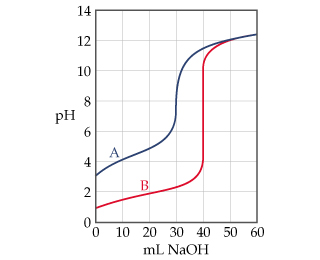# Problem: The graph below shows the titration curves for two monoprotic acids.What is the approximate pH at the equivalence point of each titration?

###### FREE Expert Solution

We are asked to estimate the pH at the equivalence point for monoprotic acid A and B from the titration curve.

titration curve is a graphical representation of the pH of a solution during a titration

The figure below shows the titration of two monoprotic acids A and B.95% (115 ratings)###### Problem Details

The graph below shows the titration curves for two monoprotic acids.What is the approximate pH at the equivalence point of each titration?